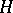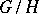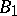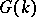# Borel subgroup

(diff) ← Older revision | Latest revision (diff) | Newer revision → (diff)
A maximal connected solvable algebraic subgroup of a linear algebraic group. Thus, for instance, the subgroup of all non-singular upper-triangular matrices is a Borel subgroup in the general linear group. A. Borel  was the first to carry out a systematic study of maximal connected solvable subgroups of algebraic groups. Borel subgroups can be characterized as minimal parabolic subgroups, i.e. algebraic subgroupsof the groupfor which the quotient varietyis projective. All Borel subgroups ofare conjugate and, if the Borel subgroups,and the groupare defined over a field,andare conjugate by an element of. The intersection of any two Borel subgroups of a groupcontains a maximal torus of; if this intersection is a maximal torus, such Borel subgroups are said to be opposite. Opposite Borel subgroups exist inif and only ifis a reductive group. Ifis connected, it is the union of all its Borel subgroups, and any parabolic subgroup coincides with its normalizer in. In such a case a Borel subgroup is maximal among all (and not only algebraic and connected) solvable subgroups of. Nevertheless, maximal solvable subgroups inwhich are not Borel subgroups usually exist. The commutator subgroup of a Borel subgroupcoincides with its unipotent part, while the normalizer ofincoincides with. If the characteristic of the ground field is 0, andis the Lie algebra of, then the subalgebraofwhich is the Lie algebra of the Borel subgroupofis often referred to as a Borel subalgebra in. The Borel subalgebras inare its maximal solvable subalgebras. Ifis defined over an arbitrary field, the parabolic subgroups which are defined overand are minimal for this property, play a role in the theory of algebraic groups oversimilar to that of the Borel groups. For example, two such parabolic subgroups are conjugate by an element of.